# Root equations

## Summary and examples

An equation with one or more root (or radical) functions is called a root or radical equation. These maybe root functions of any power, but in most cases they concern square roots. In this topic we just look at square root equations.

A square root equation can easily be solved if it has or can be deduced to the form: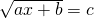Of course the following conditions have to be satisfied:

1.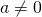because otherwise there is no equation.

2. The expression under the root sign has to be greater than or equal to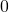, so: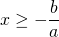3. A square root is always greater than or equal to, so: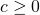When the equation has been deduced to the form:you can get the solution by squaring both sides of the equation: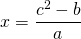When we have found the solution, we have to check whether it satisfies the conditions 2 and 3. In other words, the solution has to satisfy the original equation.

##### Example 1

Solve: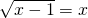The following conditions have to be satisfied: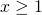and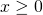so:We can square both sides of the equation: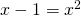This is a quadratic equation which has no solutions because the discriminant is less than: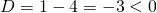##### Example 2

Solve: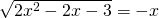We can follow two directions. Firstly, we can determine for which values ofabove equation is defined. In that case, the expression under the root sign has to be greater than or equal to zero, and also the right-hand side has to be greater than or equal to. This requires quite a few calculations. We also can first try to calculate the solutions and then afterwards see which of the solutions satisfy the equation. Here we choose the second approach.
We square both members of the equation and get: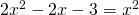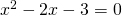We can solve this quadratic equation by factorization: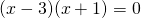resulting in the solutions: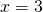or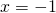We now examine whether these solutions also satisfy the original equation. After substitution we come to the conclusion that the first solution does not satisfy the equation and the second solution does: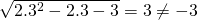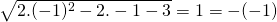##### Example 3

Solve: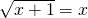The following conditions have to be satisfied: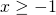andso:Squaring both sides of the equation yields: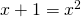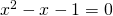This is a quadratic equation which has two different solutions because the discriminant is greater than: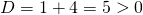The solutions of the equation are: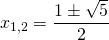The conditions above only allow positive solutions and thus the solution of the original equation is: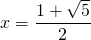##### Example 4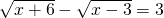For the expressions under the root sign the following conditions have to be satisfied: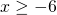and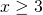so:We square both sides of the equation and get: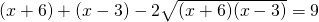After some calculations we get:Squaring both sides again yields: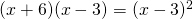This solution satisfies the conditions and therefore is a solution of the original equation.

0